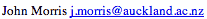Data Structures and Algorithms 12 Fast Fourier Transforms

Fourier transforms have wide application in scientific and engineering problems, for example, they are extensively used in signal processing to transform a signal from the time domain to the frequency domain.

Here, we will use them to generate an efficient solution to an apparently unrelated problem - that of multiplying two polynomials. Apart from demonstrating how the Fast Fourier Transform (FFT) algorithm calculates a Discrete Fourier Transform and deriving its time complexity, this approach is designed to reinforce the following points:

• 'Better' solutions are known to many problems for which, intuitively, it would not appear possible to find a better solution.
• As a consequence, unless you have read extensively in any problem area already, you should consult the literature before attempting to solve any numerical or data processing problem presented to you.

Because of the limitations of HTML in handling mathematical equations, the notes for this section were prepared with LaTeX and are available as a PostScript file.

©, 1998